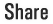# Accessing SQL parameters and its values in DQL 2 in Symfony 2

Hi All,

When you are executing a DQL there must be situation where you might need to see the parameters and its values for the underlying SQL. Usually Doctrine queries are prepared statements and you will not be able to see the values which pass through the query. Prepared statement works in the following way,

1. Sending the statement,
2. Sending the parameters,
3. And executing the prepared statement.

So, what you see when you get the SQL query is something similar to this,

` SELECT * FROM some_table WHERE column1 = ? AND column2 = ?`

So today, I am going to show you a workaround to get the parameters (such as column1, column2 and so on…) and most importantly get the respective values for those parameters. This might not be the best available solution for Doctrine users to extract the parameters and values, but definitely a worthy solution. Most importantly its easy 🙂

Let me start by giving you a DQL example first. (For the testing, I am running following code inside a Controller in Symfony 2)

```\$em = \$this-&gt;getDoctrine()-&gt;getManager();
\$result = \$em-&gt;createQueryBuilder()
-&gt;select('c')
-&gt;from(‘ACMETestBundle:Contact’, ‘c’)
-&gt;where(‘c.first_name = :fName’)
-&gt;setParameter(‘fName’, 'Anjana')
-&gt;andWhere(‘c.last_name = :lName’)
-&gt;setParameter(‘lName’, 'Silva')
-&gt;getQuery();
To get the SQL of the above query we can use \$result->getSQL(); (We all know that). The result will be,
SELECT * FROM contact AS c0_ WHERE c0_.first_name = ? AND c0_.last_name = ?
Now let’s see how to bring the parameters and it’s values in the above SQL/DQL. To do that I am going to iterate through \$result->getSQL()->getParameters(); and assign parameters and values to an array like this,
\$result = \$result-&gt;getSQL()-&gt;getParameters();
\$param_values_array = array();
foreach (\$result as \$k =&gt; \$v){
\$param_values_array[] = array(
'name' =&gt; \$v-&gt;getName(),
'value' =&gt; \$v-&gt;getValue()
);
}
The above will results this,
array[
 =&gt; array[
'name' =&gt; 'first_name',
'value' =&gt; 'Anjana'
],
 =&gt; array[
'name' =&gt; 'last_name',
'value' =&gt; 'Silva'
]
]
Really simple isn’t it.? Now you have an array fully loaded with it’s parameter names and values 🙂
By the way, one more thing to remember in here. For an instance if you have a SQL with WHERE IN, you have to re-iterate through the \$v in above example. Let’s take a DQL like this for better understanding,
\$em = \$this-&gt;getDoctrine()-&gt;getManager();
\$result = \$em-&gt;createQueryBuilder()
-&gt;select('c')
-&gt;from(‘ACMETestBundle:Contact’, ‘c’)
-&gt;where(‘c.first_name = :fName’)
-&gt;setParameter(‘fName’, 'Anjana')
-&gt;andWhere(‘c.last_name = :lName’)
-&gt;setParameter(‘lName’, 'Silva')
-&gt;andWhere(‘c.title IN (:Title)’)
-&gt;setParameter(‘Title’, array('Mr', 'Dr', 'Mast', 'Hon', 'Ven'))
-&gt;getQuery();
The above will produce an SQL like this,
SELECT * FROM contact AS c0_ WHERE c0_.first_name = ? AND c0_.last_name = ? AND c0_title IN (?)
To get the values of the Title which is an array, you need to slightly optimize the above function like this,
\$result = \$result-&gt;getSQL()-&gt;getParameters();
\$param_values_array = array();
foreach (\$result as \$k =&gt; \$v){
\$tmp = \$v-&gt;getValue();
if(is_array(\$tmp)){
\$tmp_array = array();
foreach(\$tmp as \$k1 =&gt; \$v1){
\$tmp_array[] = \$v1;
}
\$param_values_array[] = array(
'name' =&gt; \$v1-&gt;getName(),
'value' =&gt; tmp_array
);
}else{
\$param_values_array[] = array(
'name' =&gt; \$v-&gt;getName(),
'value' =&gt; \$v-&gt;getValue()
);
}
}
The above will results this,
array[
 =&gt; array[
'name' =&gt; 'first_name',
'value' =&gt; 'Anjana'
],
 =&gt; array[
'name' =&gt; 'last_name',
'value' =&gt; 'Silva'
],
 =&gt; array[
'name' =&gt; 'title',
'value' =&gt; array('Mr', 'Dr', 'Mast', 'Hon', 'Ven')
]
]
So this is how you deal with a SQL/ DQL with WHERE IN.
Well, this will wrap up today’s post. I hope you enjoyed the post.
Bye for now 🙂var hupso_services_t=new Array("Twitter","Facebook","Google Plus","Linkedin","StumbleUpon","Digg","Reddit","Bebo","Delicious"); var hupso_toolbar_size_t="medium";var hupso_url_t="";var hupso_title_t="Accessing SQL parameters and its values in DQL 2 in Symfony 2";			```